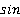### Create an Account

Home / Questions / 1 Determine the percent dynamic error of a measurement system that has an output of 3 sin ...

# 1 Determine the percent dynamic error of a measurement system that has an output of 3 sin 200t for an input of 4 sin 200t 2 Determine the attenuation reduction in units of dB decade for a

1. Determine the percent dynamic error of a measurement system that has an output of 3 sin(200t) for an input of 4 sin(200t).

2. Determine the attenuation (reduction) in units of dB/decade for a measurement system that has an output of 3(200t) for an input of 4 sin (200t) and an output of sin(2000t) for an input of 4 sin(2000t).

Jul 26 2020 View more View LessSubscribe To Get Solution